# 4 2 Relations and Functions Objectives Identify functions

• Slides: 15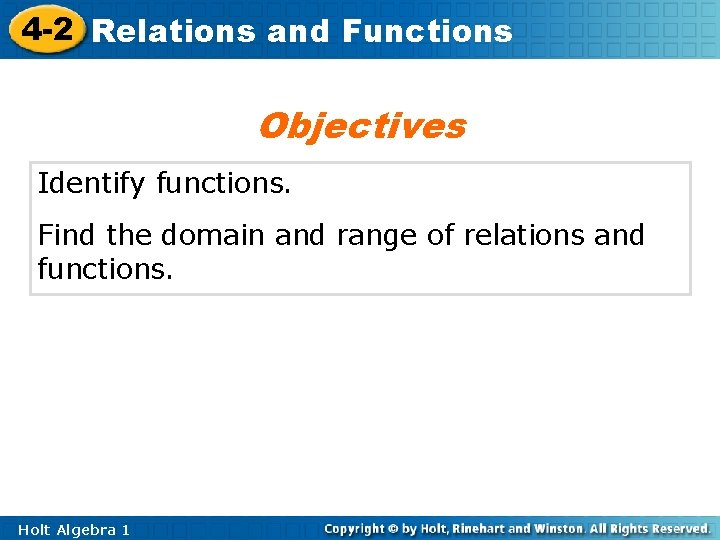4 -2 Relations and Functions Objectives Identify functions. Find the domain and range of relations and functions. Holt Algebra 1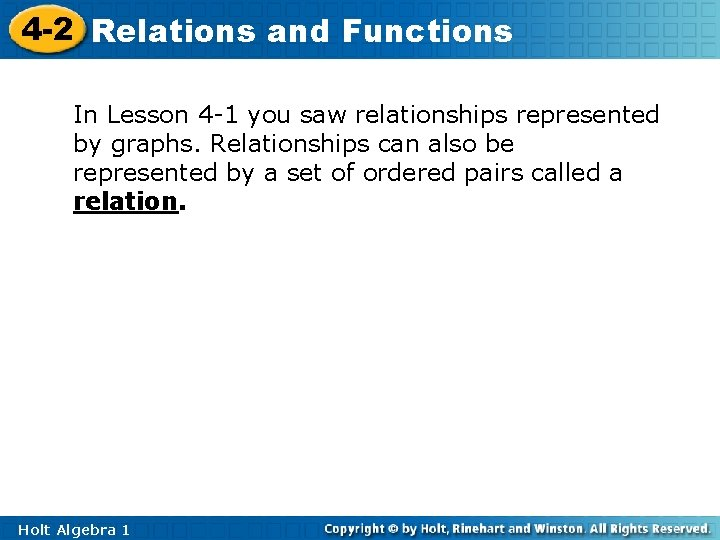4 -2 Relations and Functions In Lesson 4 -1 you saw relationships represented by graphs. Relationships can also be represented by a set of ordered pairs called a relation. Holt Algebra 14 -2 Relations and Functions Example 1: Showing Multiple Representations of Relations Express the relation {(2, 3), (4, 7), (6, 8)} as a table, as a graph, and as a mapping diagram. Table x y 2 3 4 7 6 8 Holt Algebra 1 Write all x-values under “x” and all y-values under “y”.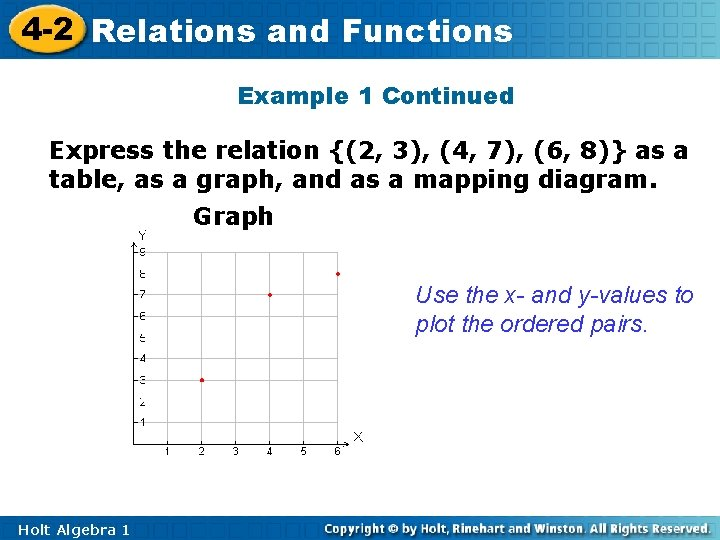4 -2 Relations and Functions Example 1 Continued Express the relation {(2, 3), (4, 7), (6, 8)} as a table, as a graph, and as a mapping diagram. Graph Use the x- and y-values to plot the ordered pairs. Holt Algebra 1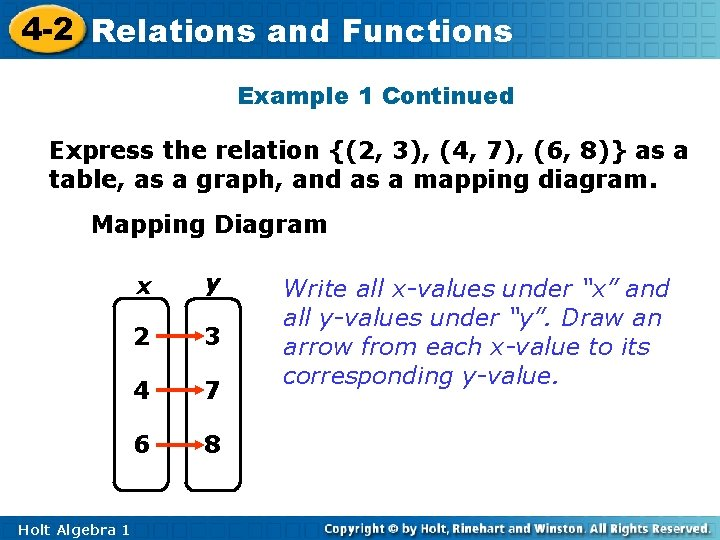4 -2 Relations and Functions Example 1 Continued Express the relation {(2, 3), (4, 7), (6, 8)} as a table, as a graph, and as a mapping diagram. Mapping Diagram Holt Algebra 1 x y 2 3 4 7 6 8 Write all x-values under “x” and all y-values under “y”. Draw an arrow from each x-value to its corresponding y-value.4 -2 Relations and Functions Check It Out! Example 1 Express the relation {(1, -3), (-2, 4), (3, 5)} as a table, as a graph, and as a mapping diagram. Table x y 1 -3 -2 4 3 5 Holt Algebra 1 Write all x-values under “x” and all y -values under “y”.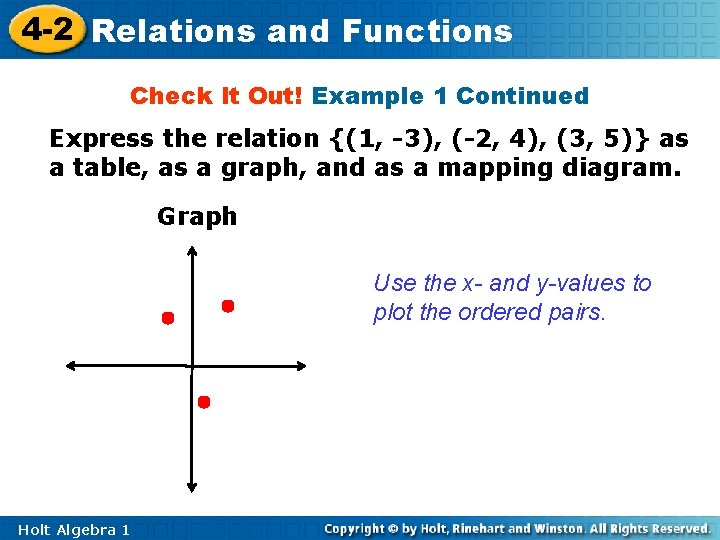4 -2 Relations and Functions Check It Out! Example 1 Continued Express the relation {(1, -3), (-2, 4), (3, 5)} as a table, as a graph, and as a mapping diagram. Graph Use the x- and y-values to plot the ordered pairs. Holt Algebra 14 -2 Relations and Functions Check It Out! Example 1 Continued Express the relation {(1, -3), (-2, 4), (3, 5)} as a table, as a graph, and as a mapping diagram. Mapping Diagram y x 1 -3 -2 4 3 5 Holt Algebra 1 Write all x-values under “x” and all y-values under “y”. Draw an arrow from each x-value to its corresponding y-value.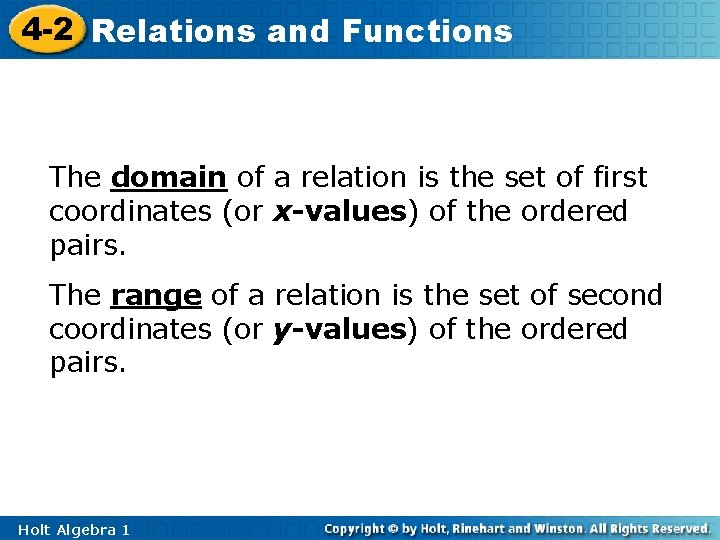4 -2 Relations and Functions The domain of a relation is the set of first coordinates (or x-values) of the ordered pairs. The range of a relation is the set of second coordinates (or y-values) of the ordered pairs. Holt Algebra 1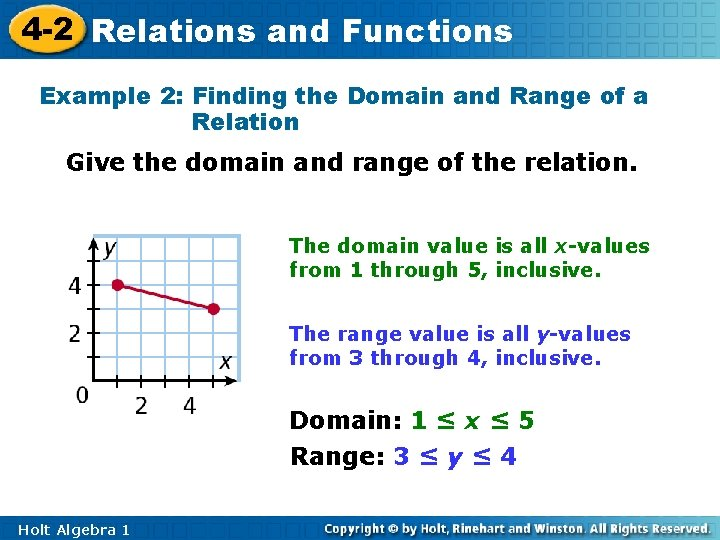4 -2 Relations and Functions Example 2: Finding the Domain and Range of a Relation Give the domain and range of the relation. The domain value is all x-values from 1 through 5, inclusive. The range value is all y-values from 3 through 4, inclusive. Domain: 1 ≤ x ≤ 5 Range: 3 ≤ y ≤ 4 Holt Algebra 1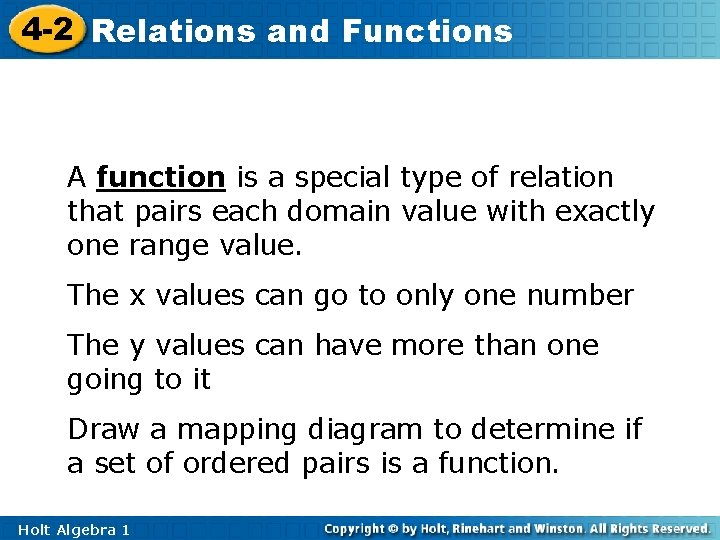4 -2 Relations and Functions A function is a special type of relation that pairs each domain value with exactly one range value. The x values can go to only one number The y values can have more than one going to it Draw a mapping diagram to determine if a set of ordered pairs is a function. Holt Algebra 14 -2 Relations and Functions Example 3 A: Identifying Functions Give the domain and range of the relation. Tell whether the relation is a function. Explain. {(3, – 2), (5, – 1), (4, 0), (3, 1)} D: {3, 5, 4} R: {– 2, – 1, 0, 1} Even though 3 is in the domain twice, it is written only once when you are giving the domain. The relation is not a function. Each domain value does not have exactly one range value. The domain value 3 is paired with the range values – 2 and 1. Holt Algebra 1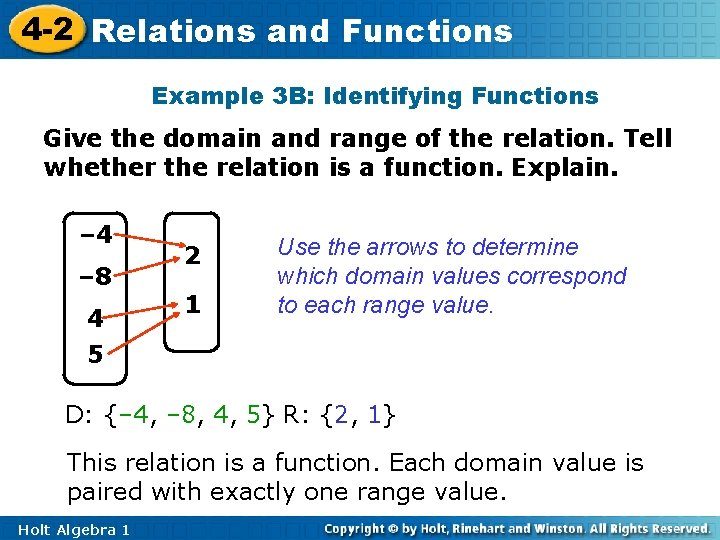4 -2 Relations and Functions Example 3 B: Identifying Functions Give the domain and range of the relation. Tell whether the relation is a function. Explain. – 4 – 8 4 5 2 1 Use the arrows to determine which domain values correspond to each range value. D: {– 4, – 8, 4, 5} R: {2, 1} This relation is a function. Each domain value is paired with exactly one range value. Holt Algebra 14 -2 Relations and Functions Lesson Quiz: Part III 3. Give the domain and range of the relation. Tell whether the relation is a function. Explain. D: {5, 10, 15}; R: {2, 4, 6, 8}; The relation is not a function since 5 is paired with 2 and 4. Holt Algebra 1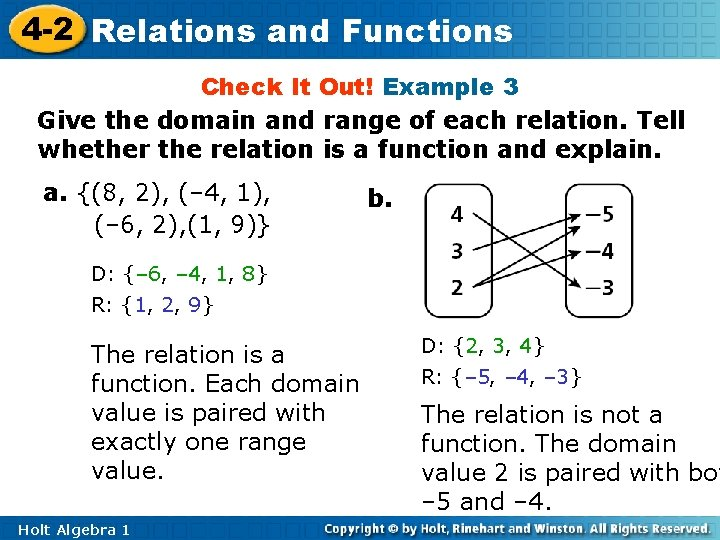4 -2 Relations and Functions Check It Out! Example 3 Give the domain and range of each relation. Tell whether the relation is a function and explain. a. {(8, 2), (– 4, 1), (– 6, 2), (1, 9)} b. D: {– 6, – 4, 1, 8} R: {1, 2, 9} The relation is a function. Each domain value is paired with exactly one range value. Holt Algebra 1 D: {2, 3, 4} R: {– 5, – 4, – 3} The relation is not a function. The domain value 2 is paired with bot – 5 and – 4.